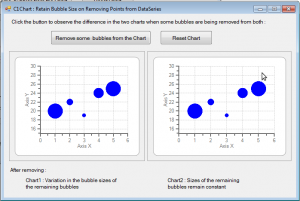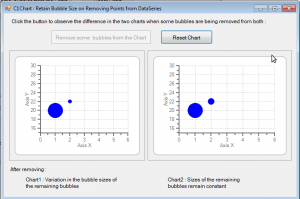﻿ C1Chart : Retain Bubble Size on Removing Points from DataSeries# C1Chart : Retain Bubble Size on Removing Points from DataSeries

C1Chart for Winforms provides a ChartType called Bubble Chart which is used to represent an additional data value at each point by changing its size. It combines two independent values to supply both the point y value and the point sizes through y1 values. When some of the bubbles are being removed from the Bubble chart the sizes of the other bubbles do not get affected until & unless the removed bubbles do not constitute of the max & min y1 data values. But, if the removed bubbles contain the max or min y1 data points then variation in the sizes of the remaining bubbles is observed. This blog explains how to control this behavior by providing a workaround, so that the remaining bubbles maintain their original sizes. We'll consider two charts here. One chart will display the variation followed by second chart which implements the workaround.

# Detailed Implementation

Set the ChartType for both the charts as Bubble & populate them with data.

``````private void BubbleChartfrm_Load(object sender, EventArgs e)
{
c1Chart1.ChartGroups.ChartType = Chart2DTypeEnum.Bubble;
c1Chart2.ChartGroups.ChartType = Chart2DTypeEnum.Bubble;
}

//Populate Charts
{
int[] x = { 1, 2, 3, 4, 5 };
int[] y = { 20, 22, 19, 24, 25 };
int[] y1 = { 19, 12, 10, 15, 19 };

chart.ChartGroups.ChartData.SeriesList.Clear();
ChartDataSeries cds = new ChartDataSeries();
cds.LineStyle.Pattern = LinePatternEnum.None;
cds.SymbolStyle.Shape = SymbolShapeEnum.Dot;
cds.SymbolStyle.Color = Color.Blue;

// Populate the chart with data
cds.X.CopyDataIn(x);
cds.Y.CopyDataIn(y);
cds.Y1.CopyDataIn(y1);

// Set the Max & Min properties for the chart
chart.ChartArea.AxisX.Max = 6;
chart.ChartArea.AxisX.Min = 0;
chart.ChartArea.AxisY.Max = 30;
chart.ChartArea.AxisY.Min = 15;
}
``````

Now remove some of the bubbles from both the charts , say, on the Click of a Button. As soon as the bubbles are removed, the sizes of the remaining bubbles in both the charts variate. However, this behavior is correct for a bubble chart. Think of the ChartGroup.Bubble object MinimumSize and MaximumSize properties as min and max values for a third axis (the Y1 data). MinimumSize specifies the smallest possible size of a bubble (axis min) and MaximumSize specifies the largest possible size of a bubble (axis max). All the bubble sizes then fit linearly between the Min and Max sizes. For example, if your Y1 values are 10, 15 and 30, and your MinimumSize = 10 pixels, and your MaximumSize = 30 pixels, then the Y1=10 bubble will be 10 pixels, the Y1=15 bubble will be 15 pixels and the Y1=30 bubble will be 30 pixels. By definition, if you only have 2 points, then the smallest will be the MinimumSize and the largest will be the MaximumSize. Now in the current chart data, the minimum value is removed from the data, and maximum value is retained. Therefore the maximum value bubble does not change size and the new minimum value is assigned to the MinimumSize after the button click. So, if you want all of the bubbles to remain the same size when adding or removing data, you need to maintain the Y1 data max and min values. For the case where we are trimming data, this could be done as follows :

`````` //Remove bubbles from BubbleCharts
private void RemoveBtn_Click(object sender, EventArgs e)
{
Remove(c1Chart1);
Remove(c1Chart2);

ChartDataSeries cds = c1Chart2.ChartGroups.ChartData.SeriesList;

// find the existing min and max bubble sizes.
int miny1 = int.MaxValue, maxy1 = int.MinValue;
int[] y1 = cds.Y1.CopyDataOut(typeof(int)) as int[];
for (int i = 0; i < y1.Length; i++)
{
if (miny1 > y1[ i ]) miny1 = y1[ i ];
if (maxy1 < y1[ i ]) maxy1 = y1[ i ];
}

// introduce points out of viewable range that maintain
// max and min size ratios.
cds.PointData.Insert(0, new PointF(-1, 0));
cds.Y1.Insert(0, maxy1);
cds.PointData.Insert(0, new PointF(-2, 0));
cds.Y1.Insert(0, miny1);
c1Chart2.ChartGroups.ChartData.SeriesList.PointData.Length = 2 + 2;
RemoveBtn.Enabled = false;

}
``````

where the Remove function is as follows :

``````private void Remove(C1Chart chart)
{
// Remove last 3 datapoints from the chart also containing the minimum value in Y1
chart.ChartGroups.ChartData.SeriesList.PointData.Length = 2;
}
``````

Here are the images before and after the implementation. Before the implementation.After the implementation.Download the attached samples for complete implementation. Download Sample C# Download Sample VBGrapeCity

GrapeCity Developer Tools